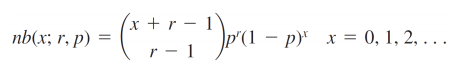# Negative Binomial Experiment / Distribution: Definition, Examples

Binomial Theorem > Negative Binomial Experiment

The negative binomial experiment is almost the same as a binomial experiment with one difference: a binomial experiment has a fixed number of trials.

If the following five conditions are true the experiment is binomial:

1. Fixed number of n trials.
2. Each trial is independent.
3. Only two outcomes are possible (Success and Failure).
4. Probability of success (p) for each trial is constant.
5. A random variable Y= the number of successes.

Example: Take a standard deck of cards, shuffle them, and choose a card. Replace the card and repeat twenty times. Y is the number of aces you draw.

The negative binomial is similar to the binomial with two differences (specifically to numbers 1 and 5 in the list above):

• The number of trials, n is not fixed.
• A random variable Y= the number of trials needed to make r successes.

Example: Take a standard deck of cards, shuffle them, and choose a card. Replace the card and repeat until you have drawn two aces. Y is the number of draws needed to draw two aces. As the number of trials isn’t fixed (i.e. you stop when you draw the second ace), this makes it a negative binomial distribution.

## What is a Negative Binomial Distribution?

A negative binomial distribution (also called the Pascal Distribution) is a discrete probability distribution for random variables in a negative binomial experiment.

The random variable is the number of repeated trials, X, that produce a certain number of successes, r. In other words, it’s the number of failures before a success. This is the main difference from the binomial distribution: with a regular binomial distribution, you’re looking at the number of successes. With a negative binomial distribution, it’s the number of failures that counts.

## Why is it called Negative Binomial?

When you hear the term negative, you might think that a positive distribution is flipped over the x-axis, making it negative. However, the “negative” part of negative binomial actually stems from the fact that one facet of the binomial distribution is reversed: in a binomial experiment, you count the number of Successes in a fixed number of trials; in the above example, you’re counting how many aces you draw. In a negative binomial experiment, you’re counting the Failures, or how many cards it takes you to pick two aces.

## The Negative Binomial Formula

Probability:
b*(x; r, P) = x-1Cr-1 * Pr * (1 – P)x – r
where x=number of trials
r = Successes

Mean:
μ = r / P
where r is the number of trials
P=probability of success for any trial

## Solving Negative Binomial Experiment Problems

The pmf for the negative binomial distribution is:Where:
r is the number of successes and
p = the probability of success.

Sample question: You are surveying people exiting from a polling booth and asking them if they voted independent. The probability (p) that a person voted independent is 20%. What is the probability that 15 people must be asked before you can find 5 people who voted independent?

Step 1: Find p, r and X.
We are given (in the question) that p = 20%(.2) and r = 5. The number of failures, X, is 15 – 5 = 10.

Step 3: Solve. The first part (14 over 4) is a combination (use our combinations calculator to find 14 choose 4).
1001*.25*.810 = 0.034.

The probability you’ll have to ask 15 people to get 5 votes for independent is .034, or 3.4%.

The geometric distribution is a special case of the negative binomial distribution.

CITE THIS AS:
Stephanie Glen. "Negative Binomial Experiment / Distribution: Definition, Examples" From StatisticsHowTo.com: Elementary Statistics for the rest of us! https://www.statisticshowto.com/negative-binomial-experiment/